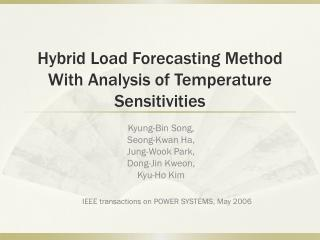Download PresentationHybrid Load Forecasting Method With Analysis of Temperature Sensitivities

# Hybrid Load Forecasting Method With Analysis of Temperature Sensitivities - PowerPoint PPT Presentation

Hybrid Load Forecasting Method With Analysis of Temperature Sensitivities. Kyung-Bin Song, Seong -Kwan Ha, Jung- Wook Park, Dong-Jin Kweon , Kyu -Ho Kim. IEEE transactions on POWER SYSTEMS, May 2006. Outline. Introduction Hybrid Short-Term Load Forecasting (STLF) MethodsI am the owner, or an agent authorized to act on behalf of the owner, of the copyrighted work described.
Download Presentation## Hybrid Load Forecasting Method With Analysis of Temperature Sensitivities

Download Policy: Content on the Website is provided to you AS IS for your information and personal use and may not be sold / licensed / shared on other websites without getting consent from its author.While downloading, if for some reason you are not able to download a presentation, the publisher may have deleted the file from their server.

- - - - - - - - - - - - - - - - - - - - - - - - - - E N D - - - - - - - - - - - - - - - - - - - - - - - - - -
Presentation Transcript
1. Hybrid Load Forecasting Method With Analysis of Temperature Sensitivities Kyung-Bin Song, Seong-Kwan Ha, Jung-Wook Park, Dong-Jin Kweon, Kyu-Ho Kim IEEE transactions on POWER SYSTEMS, May 2006

2. Outline • Introduction • Hybrid Short-Term Load Forecasting (STLF) • Methods • Exponential Smoothing • Fuzzy Linear Regression • Temperature Sensitivities • Results • Conclusion

3. Introduction Conventional methods on STLF are not suitable for higher load forecasting errors in some particular time zones, like weekends. So intelligence techniques like fuzzy and neural network are recently as an alternative in forecasting. In this paper, a new hybrid load forecasting consist of fuzzy linear regression and the general exponential smoothing with analysis of temperature sensitivities is proposed. Each has their usage on different situation.

4. Hybrid Short-Term Load Forecasting General patterns of weekdays are almost identical, but on weekends and Mondays are depend on consumers lifestyle. Especially, the load patterns of a summer season are strongly affected by the temperature. In this paper, Mondays and weekends are processed together for better accuracy. And hybrid STLF with temperature is used in summer.

5. Flow chart

6. Methods – Exponential Smoothing General exponential smoothing is developed to provide load forecasting of weekdays without regarding to weather conditions and special vacations. In other words, these methods only use in spring, fall and winter. A smoothing time series model :F is the forecasted value, X is real value, N is the number of observation data

7. Methods – Exponential Smoothing Assume the observation data varies slowly, using time series model : substitute with for general : Using maximum load during three days prior to the predicted day, final equation :

8. Methods – Exponential Smoothing example - Predicted day : March 26(Tue)Three observation days maximum load : March 25(Mon), 22(Fri), 21(Thu) : 1/3the equation of forecasted load:

9. Normalized The average normalized value for 24 hourly loads = , are the maximum and hourly load on previous same day as the predicted day. The hourly load on the predicted day : This operation attached to all the methods

10. Method - Fuzzy linear regression Linear regression is a statistical method to model the relationship between two variables by fitting a linear equation to observed data Fuzzy linear regression model can expressed as :All variables are fuzzy numbers, and are fuzzy addition and multiplication Triangular fuzzy number

11. Method - Fuzzy linear regression • Fuzzy number • addition • multiplication • For simplicity, assume all the variables are symmetric fuzzy numbers, then , ,, , and the result of fuzzy linear regression analysis using shape preserving operation :

12. Method – temperature sensitivities

13. Method – temperature sensitivities Step 1 : Select 3 years highest temperature/load correlation coefficient input data among past 5 years of predicted year.Step 2 : Divide predicted period into two parts, July and August, which have different temperature sensitivities.Step 3 : calculate variation of temperature between the predicted day and one previous day and are maximum temperature of predicted day and previous day

14. Method – temperature sensitivities Step 4 : calculate the variation of load and are the maximum loadsStep 5 : compute the temperature sensitivities using and

15. Method – temperature sensitivities Step 6 : check the signs of in July and August, and compute the average of each positive and negative valueStep 7 : forecast the maximum load on predicted day, is the forecasted maximum load in the one day before predicted day

16. Results The algorithm is applied to forecast a week(different seasons) of 1996 Comparative algorithm is the “top-down developed method”, called Load Forecasting Engineering System(LOFES), by Korea Electric Power Research Institute(KEPRI), which use time-domain regressive analysis with past load profile and weather data.

17. Results March 26 to April 1(spring)

18. Results October 29 to November 4(fall)

19. Results July 9 to July 15

20. Conclusion This paper proposed a new hybrid short-term load forecasting algorithm. It combines exponential smoothing, fuzzy linear regression and temperature sensitivities. The temperature sensitivity is a new approach and it actually improves the load forecast accuracy in summer